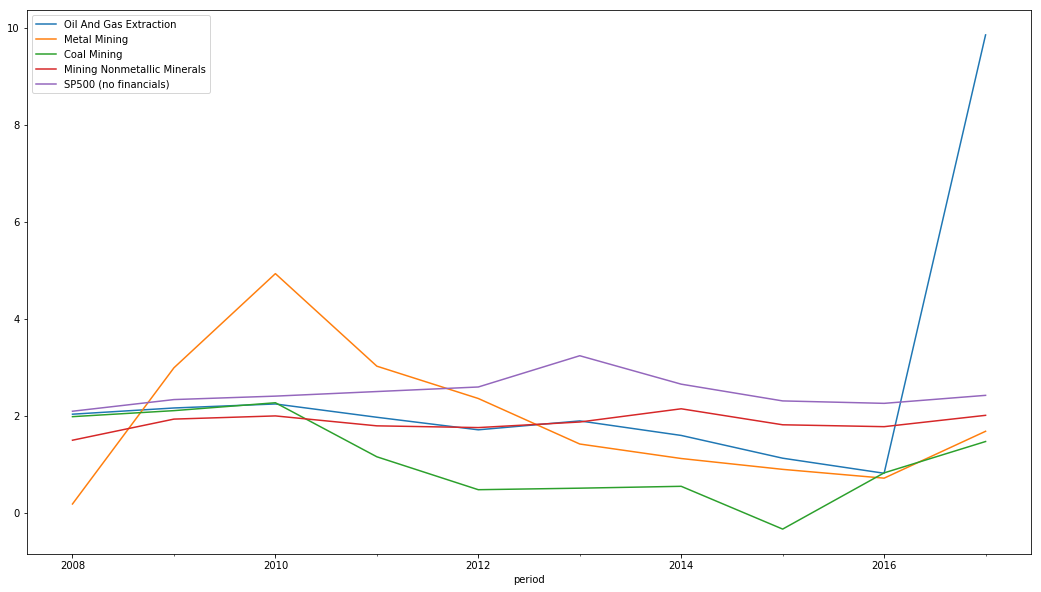# Predicting Bankruptcy in the mining industry with Altman's Z-score and the Calcbench API¶

Edward Altman's Z-score is a formula for predicting corporate bankruptcy. Below we plot the change in industry aggregate Z-scores for mining companies. A Z-score above 2.99 is considered "safe", between 1.81 and 2.99 is a "gray" zone, below 1.81 is the "distress" zone. The troubles in the coal and metal mining industry are clearly illustrated.

Altman's Z-score @ Wikipedia

To replicate the below sign up for a Calcbench account, install the Calcbench Python API client from here and download this note book from github.

In :
import calcbench as cb
import pandas as pd
pd.options.display.max_rows = 1000
%pylab inline

Populating the interactive namespace from numpy and matplotlib

In :
z_score_metrics = ['CurrentAssets',
'CurrentLiabilities',
'Assets',
'RetainedEarnings',
'EBIT',
'MarketCapAtEndOfPeriod',
'Liabilities',
'Revenue']

In :
SIC_codes = {
"Oil And Gas Extraction" : 1300,
"Metal Mining" : 1000,
"Coal Mining" : 1200,
"Mining Nonmetallic Minerals" : 1400,
}

In :
def peer_group_z_score(peer_group):
peer_group = peer_group[(peer_group.ticker != 'GMC') & (peer_group.ticker != 'PPI')] #GMC's marketvalue is off
z_score_data = cb.normalized_dataframe(company_identifiers=list(peer_group.ticker),
metrics=z_score_metrics,
start_year=2008, start_period=0,
end_year=2017, end_period=0)
aggregate_data = z_score_data.sum(level=, axis=1)
return compute_z_score(aggregate_data), z_score_data

In :
def compute_z_score(inputs):
#from https://github.com/calcbench/notebooks/blob/master/z-score.ipynb
working_capital = inputs['CurrentAssets'] - inputs['CurrentLiabilities']

z_score = (1.2 * (working_capital / inputs['Assets']) +
1.4 * (inputs['RetainedEarnings'] / inputs['Assets']) +
3.3 * (inputs['EBIT'] / inputs['Assets']) +
0.6 * (inputs['MarketCapAtEndOfPeriod'] / inputs['Liabilities']) +
.99 * (inputs['Revenue'] / inputs['Assets']))

return z_score

In :
peer_groups = [(industry, cb.companies(SIC_codes=[SIC_code])) for industry, SIC_code in SIC_codes.items()]
sp500 = cb.companies(index="SP500")
sp500_no_financials = sp500[sp500.sic_code & ((sp500.sic_code < 6000) | (sp500.sic_code >= 7000))] # There is a different z-score formulas for financials.
peer_groups.append(("SP500 (no financials)", sp500_no_financials))
industry_z_scores = [(industry, peer_group_z_score(peer_group)) for industry, peer_group in peer_groups]
z_scores = pd.DataFrame.from_items(industry_z_scores)

c:\users\andrew kittredge\appdata\local\programs\python\python37-32\lib\site-packages\ipykernel_launcher.py:6: FutureWarning: from_items is deprecated. Please use DataFrame.from_dict(dict(items), ...) instead. DataFrame.from_dict(OrderedDict(items)) may be used to preserve the key order.


In :
z_scores.plot(figsize=(18, 10))

Out:
<matplotlib.axes._subplots.AxesSubplot at 0x96f030>In :
z_score, data = peer_group_z_score(peer_groups)

In :
compute_z_score(data.swaplevel(0, 1, 1).ACI)

Out:
period
2008         NaN
2009    1.496238
2010    2.372645
2011    0.872310
2012    0.393262
2013    0.211907
2014    0.247459
2015         NaN
Freq: A-DEC, dtype: float64
In [ ]: